# Python Program to Compare Two Strings

In this article, we've created some programs in Python to compare two strings entered by user at run-time. Here are the list of programs:

• Compare Two Strings in Character-by-Character manner
• Compare Two Strings using for loop
• Using list
• Using == operator
• Using function
• Using class

## Compare Two Strings Character-by-Character

To compare two strings in Python, you have to ask from user to enter any two strings, then check whether the value of two strings are equal or not in character-by-character manner as shown in the program given below:

```print("Enter the First String: ")
strOne = input()
print("Enter the Second String: ")
strTwo = input()

lenOne = len(strOne)
lenTwo = len(strTwo)

if lenOne==lenTwo:
i = 0
chk = 0
while i<lenOne:
if strOne[i] != strTwo[i]:
chk = 1
break
i = i+1
if chk==0:
print("\nStrings are Equal")
else:
print("\nStrings are Not Equal")
else:
print("\nStrings are Not Equal")```

Here is the sample run of above Python program to demonstrate its output. This is the initial output: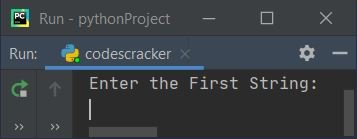Now enter the first string say codescracker and press `ENTER` key, again enter the second string say codescracker and again press `ENTER` key to compare two strings and print the message whether both strings entered by user are equal or not as shown in the snapshot given below: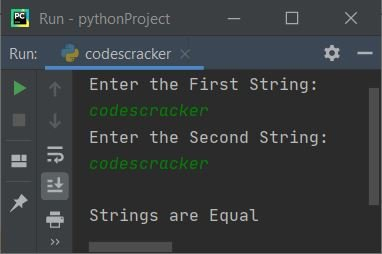Here is another sample run with user input, codes as first and cracker as second string: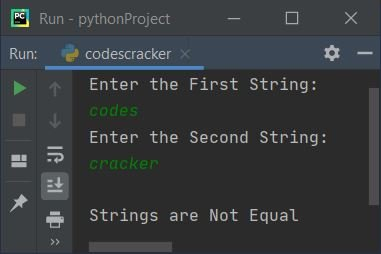#### Previous Program Explained

The following two statements:

```lenOne = len(strOne)
lenTwo = len(strTwo)```

is used to initialize the length of string stored in strOne to lenOne. Similarly, the length of second string gets initialized to lenTwo.

For example, if user enters first string as codescracker and second string as same as first. Then the dry run of above program goes like:

• Through above two statements, lenOne=12 and lenTwo=12
• Now the condition, lenOne==lenTwo evaluates to be true, therefore program flow goes in the body of if block. There, i=0, chk=0. And the condition i<lenOne or 0<12 evaluates to be true, therefore program flow goes inside the while loop
• Inside the while loop, the condition strOne[i] != strTwo[i] or strOne != strTwo or c != c evaluates to be false, therefore program flow does not goes inside the if's block
• Therefore i+1 or 0+1 or 1 gets initialized to i. So i=1 and the condition i<lenOne again gets evaluated with new value of i. This time also the condition i<lenOne or 1<12 evaluates to be true, therefore program flow again goes inside the loop and compares the character at corresponding index of both strings
• If any mismatch found, then we've initialized 1 to chk and using break keyword, the execution of while loop gets terminated. In this way, after exiting from the loop, either when the condition of while loop evaluates to be false, or using break keyword, we've checked the value of chk
• That is, if it contains 0 then program flow never goes inside the if block (inside while loop). That means, no any mismatch found. Otherwise if it contains 1, means a mismatch found
• In this way, the string gets compared in character-by-character manner

#### Modified Version of Previous Program

This program uses end to skip printing of an automatic newline using print(). The str() method is used to convert any type of value to a string type. And \" is used to print ". Rest of the things are similar to previous program.

```print("Enter Two Strings: ", end="")
sOne = input()
sTwo = input()

sOne = str(sOne)
sTwo = str(sTwo)

lOne = len(sOne)
lTwo = len(sTwo)

if lOne==lTwo:
i = 0
chk = 0
while i<lOne:
if sOne[i] != sTwo[i]:
chk = 1
break
i = i+1
if chk==0:
print("\n\"" +sOne+ "\" = \"" +sTwo+ "\"")
else:
print("\n\"" +sOne+ "\" != \"" +sTwo+ "\"")
else:
print("\n\"" +sOne+ "\" != \"" +sTwo+ "\"")```

Here is its sample run with user input Welcome to CodesCracker as first and This is CodesCracker as second string: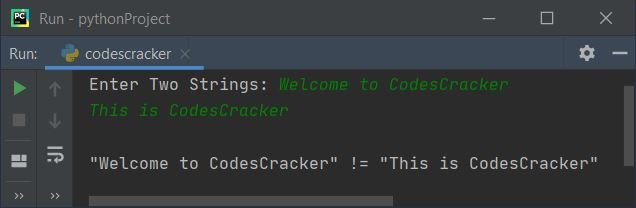## Compare Two Strings using for Loop

This program uses for loop instead of while loop to compare two strings in character-by-character manner. The range() method returns a sequence of values. By default, the value starts with 0 and increments by 1. It stops before a number specified as its argument:

```print("Enter Two Strings: ", end="")
sOne = input()
sTwo = input()

sOne = str(sOne)
sTwo = str(sTwo)
lOne = len(sOne)
lTwo = len(sTwo)

if lOne==lTwo:
chk = 0
for i in range(lOne):
if sOne[i] != sTwo[i]:
chk = 1
break
if chk==0:
print("\n\"" +sOne+ "\" = \"" +sTwo+ "\"")
else:
print("\n\"" +sOne+ "\" != \"" +sTwo+ "\"")
else:
print("\n\"" +sOne+ "\" != \"" +sTwo+ "\"")```

This program produces the same output as of previous program.

## Compare Two Strings using List

This program uses list to compare two strings entered by user. That is, we've initialized the first string to a list named sOneList and checked the second string stored in sTwo using in, whether it is available in the list or not as shown in the program given here:

```print("Enter Two Strings: ", end="")
sOne = input()
sTwo = input()

sOneList = []
sOneList = sOne

if sTwo in sOneList:
print("\nEqual Strings")
else:
print("\nUnequal Strings")```

Here is its sample run with user input python as first and python as second string: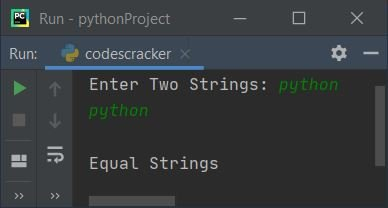## Compare Two Strings Directly using ==

This is the shortest program to compare two strings in Python. This program uses == operator to do the job.

```print("Enter Two Strings: ", end="")
sOne = input()
sTwo = input()

if sOne==sTwo:
print("\nEqual Strings")
else:
print("\nUnequal Strings")```

## Compare Two Strings using Function

This program uses a user-defined function named compareStrings() to compare two strings. That is, this function receives both strings as its argument and returns 1 if both strings are equal using == operator

```def compareStrings(x, y):
if x==y:
return 1

print("Enter Two Strings: ", end="")
sOne = input()
sTwo = input()

chk = compareStrings(sOne, sTwo)
if chk==1:
print("\nEqual Strings")
else:
print("\nUnequal Strings")```

## Compare Two Strings using Class

This program uses class, an object-oriented feature of Python to do the same job. That is to compare two given strings by user:

```class CodesCracker:
def compareStrings(self, x, y):
if x==y:
return 1

print("Enter Two Strings: ", end="")
sOne = input()
sTwo = input()

obj = CodesCracker()
chk = obj.compareStrings(sOne, sTwo)
if chk==1:
print("\nEqual Strings")
else:
print("\nUnequal Strings")```

We've created an object named obj of class CodesCracker to access its member function named compareStrings() through dot (.) operator.

#### Same Program in Other Languages

Python Online Test

« Previous Program Next Program »